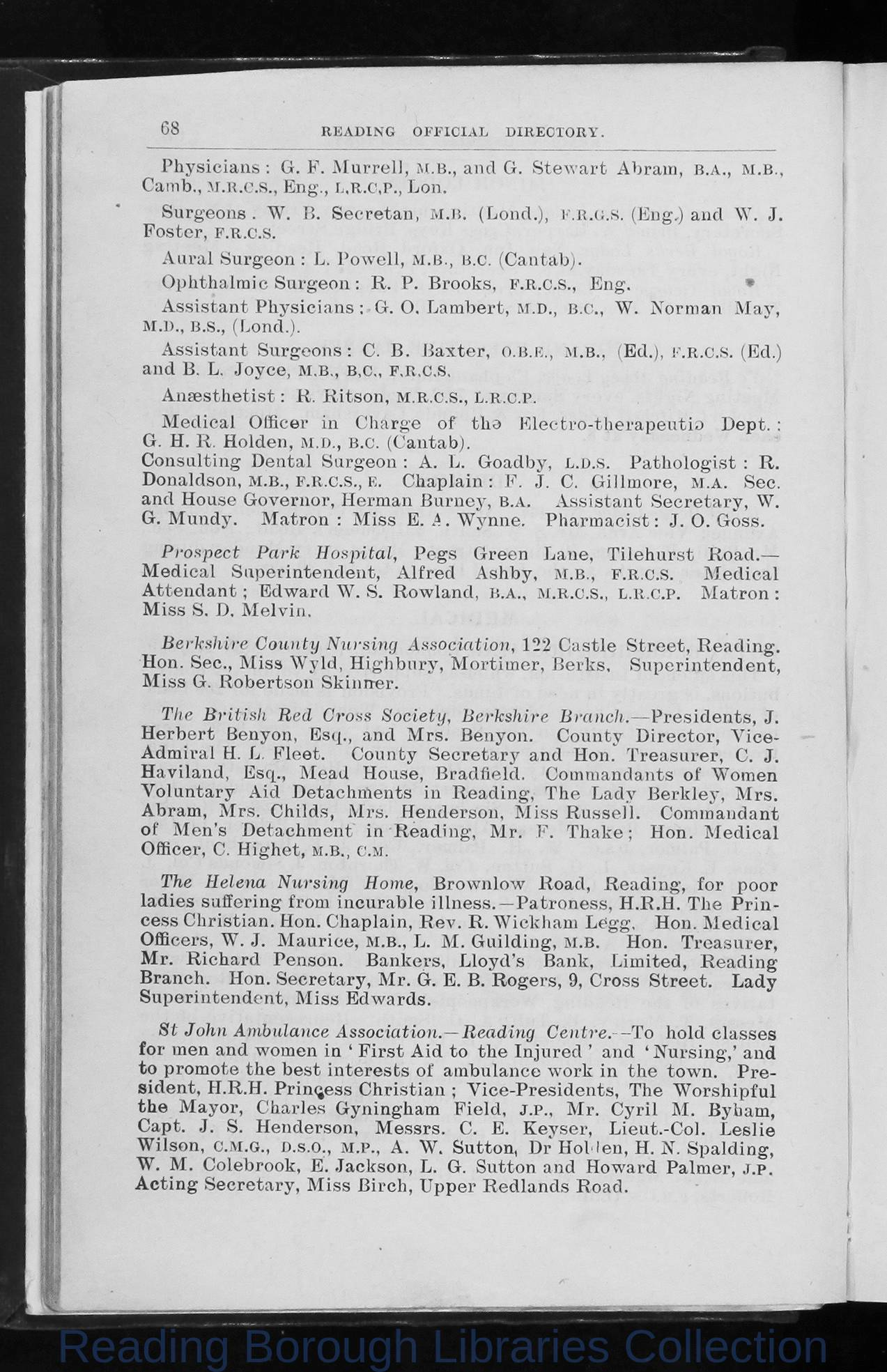## Image Details

There is no information available.

## OCR Text

68

READING OFFICIAL DIRECTORY.

P h ysic ia n s : G. F . M u rrell, M.B., and G. S t e w a r t A bram ,
Camb., M.R.G.S., Eng., l ,r .c ,p ., Lon.
S u rgeon s . W . B. S e ere ta n , M.B. (Lond.),
F o ster, f .r .c .s .

f .r . g .s .

b .a .,

M.B.,

(Eng.) and \Y. J.

A u ra l Surgeon : L. P o w ell, M.B., B.C. (Cantab).
O ph th alm ic S u r g e o n : R . P. Brooks, F.R.C.S., E n g.
*
A ss is ta n t P h ysic ia n s : G. O. Lam bert, M.D., B.C., W. Norman M ay,
M.D., B.S., (Lond.).
A ss is ta n t S u rg eo n s: C. B . B a x te r, O.B.E., M.B., (Ed.), F.R.C.S. (Ed.)
and B. L. Jo yce, M.B., B.C., F.R.C.S.
A n a esth etist: R. R itso n ,

m .r .c .s .,

L.r .c .p .

M ed ical Officer in C h arge o f tlio E lectro -th e ra p e u tio D ept. :
G. H. R . Holden, M.D., B.C. (Cantab).
C o n su ltin g D en tal Surgeon : A. L. G oadby, L.D.S. P a th o lo g is t : R .
Donaldson, M.B., F.R.C.S., E. Chaplain : F. J. C. G illm ore, m .a . Sec.
and House G overn or, Herm an B urn ey, B.A. A ss is ta n t S e c r e ta r y , W.
G. M undy. M atron : M iss E. A. W ynne. P h a r m a c is t: J. 0 . Goss.
P rospect Park Hospital, P e gs G reen Lan e, T ile h u rs t R o ad .â
M e d ical S u p erin ten d en t, A lfre d A sh by, M.B., F.R.C.S.
M e d ical
A tte n d a n t; Edw ard W. S. R ow lan d , B.A., M.R.C.S., L.R.C.P. M atro n :
M iss S. D. M elvin .
Berkshire County Nursing Association, 122 C a stle S tr e e t, R e a d in g.
Hon. Sec., M iss W yld, H igh b u ry, M ortim er, B erk s, S u p erin ten d e n t,
M iss G. R obertson Sk in n er.
The British Red Cross Society, Berkshire Branch.â P re sid e n ts, J.
H e rb ert Benyon, Esq., and M rs. Benyon. C o u n ty D ire c to r, V ic e A dm iral H. L. F le et.
C o un ty S e c r e ta r y and Hon. T rea su re r, C. J.
H aviland, Esq., M ead House, B rad field. Com m andants o f Women
V o lu n ta ry A id D etach m en ts in R ea d in g, T h e L ad y B e r k le y , M rs.
Abram , M rs. C h ild s, M rs. H enderson, M iss R u s s e ll. Comm andant
o f M en's D etach m ent in R ea d in g, M r. F. T h a k e ; Hon. M ed ical
Officer, C. H igh et, M.B., c.m.
The Helena Nursing Home, B row nlow Road, R ea d in g, fo r poor
la d ies sufferin g from in cu rab le illn e s s .â P a tro n ess, H.R.H. T he P r in Â­
ce ss C h ristian . Hon. C h ap lain , R e v . R . W ickham L c g g . Hon. M e d ic a l
Officers, W. J. M au rice, M.B., L. M . G uilding, M.B.
Hon. T re a su re r,
M r. R ich ard Penson. B an kers, L lo y d âs Bank, Lim ited, R ea d in g
B ran ch . Hon. S e c re ta ry , M r. G. E. B. R o gers, 9, C ross S tre e t. L ad y
Su p erin ten d en t, M iss Edw ards.
St John Ambulance Association.âReading C entre. - To hold classe s
for men and women in â F ir s t A id to th e Injured â and â N u rsin g,â and
to prom ote th e b e s t in te r e s ts o f am bulance w ork in th e tow n. P r e Â­
sid e n t, H.R.H. P rin cess C h ristia n ; V ic e -P resid e n ts, The W o rsh ipfu l
th e M ayor, C h arles G yningham F ield , J.P., M r. C y r il M. Bybam ,
C apt. J. S. H enderson, M essrs. C. E. K ey ser, Lieu t.-C o l. L e s lie
W ilson, c.M.G., D.s.o., M.P., A. W. Sutton, Dr HoM en, H. N. Sp ald in g,
W. M. Colebrook, E. Jackson, L. G. Sutton and H ow ard Palm er, J.p.
A c tin g S e c re ta ry , M iss B irch , U pper R ed la n d s Road.